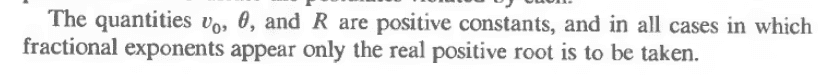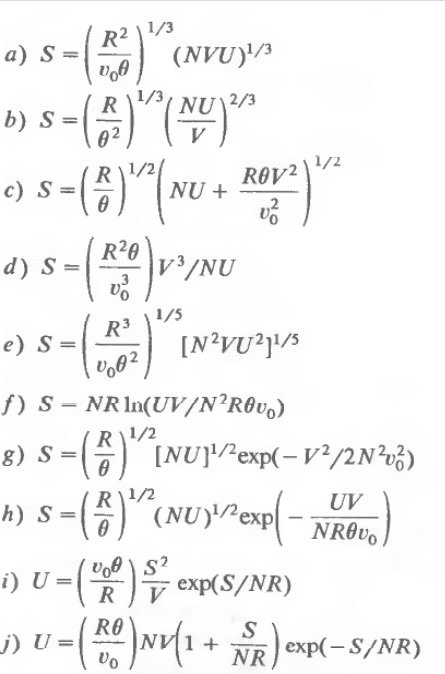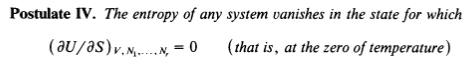# Easy entropy problem, what is the permitted entropy?

LCSphysicist
Homework Statement:
All below
Relevant Equations:
All belowThere is five physically possible entropy to exist, and five entropy which can't be real, find it all.

I could found just four entropy, what is the another?

B, H and J:
S(λU,λV,λN) ≠ λS(U,V,N)
D:
∂S/∂U < 0

what is the another?
(another or other??)

Homework Helper
Gold Member
This is a problem from Callen's textbook. Did you consider violations of Callen's Postulate IV?LCSphysicist
This is a problem from Callen's textbook. Did you consider violations of Callen's Postulate IV?

View attachment 267814
Actually i thought about it, since was the only postulate i not mentioned, however, i don't know how could i deal with this, since i don't have an equation in the options which leave me direct to the temperature.
I don't think we can say U = NfkT/2 as generally do for some cases.

Homework Helper
Gold Member
From the functional form of each relation between S and U, you can consider if there are any states where $$\left( \frac{\partial U}{\partial S} \right)_{V, N} = 0$$
If there are such states, do these states satisfy ##S = 0##?

•LCSphysicist
LCSphysicist
From the functional form of each relation between S and U, you can consider if there are any states where $$\left( \frac{\partial U}{\partial S} \right)_{V, N} = 0$$
If there are such states, do these states satisfy ##S = 0##?
Oh, i was making a confusing zzz thx